PHY294H - Lecture 42

How the human eye works

The human eye is an extraordinary optical instrument. Light is focused on the retina which consists of about 108 photoreceptors which come in two types, rods and cones. These photoreceptors are attached to 106 axons of ganglion cells. The ganglion cells make up the optic nerve which transmits impulses to the brain. The photoreceptors detect individual photons. All of the ganglions in the optic nerve have a slow steady firing rate, even in the dark. A diffuse light does not affect this rate. However an more intense directed beam of light induces a rapid increase in the firing rate of the ganglions attached to the photoreceptrs attached to the rods and cones where this light is focused (http://users.rcn.com/jkimball.ma.ultranet/BiologyPages/V/VisualProcessing.html).

Light is focused on parts of the retina by the lens. The lens is long and thin when we look at distant objects. It is short and fat when we look at close objects, e.g. when we read. In the relaxed state, the lens is long and thin. If the resting state of the lens is not thin enough, light from distant objects are is focused before the retina. This is a condition called near-sightedness and is the most frequent vision problem in the very young. In contrast if the lens cannot be made convergent enough to focus the light from close objects on the retina, then the person is far-sighted. This is the most frequent vision problem of adults, who then require reading glasses.

Angular size

In our discussion of mirrors, refracting surfaces and lenses, we have defined mangification to be M=-i/s = hi/hs. This definition is not the most useful one when considering optical instruments such as microscopes and telescopes. In these instruments the key measure of magnification is the angular size of an object or image. The angle subtended by an object, or by an image is,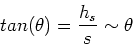(1)

In almost all optical instruments this angle is small, so we can make the approximation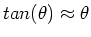.

Maximum angular size achievable with the naked eye

The way in which we can increase the angular magnification of an object, using only the naked eye, is simply to move our eyes closer to it. This is limited by the ability of the lens in our eye to focus the light from near objects onto the retina. The closest objects which can be brought into focus by adults is about 25cm, so the angular extent that the naked eye can achieve is(2)

where.

Angular magnification - Simple magnifier

The simple magnifier (magnifying glass) is a convergent lens which can assist the eye by allowing the eye to move even closer to the object of interest. Converging lenses produce a magnified, virtual, image when the object is placed inside the focal length of the lens. If the object is placed at the focal point of the lens, then the image is at infinity, and the magnification is M = -i/f = hi/hs. The angular extent of the image produced by the magnifier,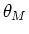, is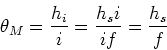(3)

We define the angular magnification,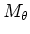by comparing this with the maximum angular size that can be achieved by the naked eye, so that,(4)

By making the focal length f much smaller than the length dmin significant magnification can be achieved.

Compound microscope

Much larger angular magnifications can be achieved using the compound microscope. This instrument is attributed to Hans and Zacharias Jansen in 1590 though they only achieved 9x magnification. In the 1700's however early compound microscopes could magnify up to about 250 times, which opened up direct observation of the world of microbiology. This was first achieved by Anton van Leeuwenhoek in 1676 who made the first direct observations of bacteria. Modern optical microscopes can achieve up to 2000x magnification, but they are ultimately limited by diffraction effects due to the wavelength of light.

The compound microscope consists of two converging lenses. The object is placed in front of an objective lens. The object is placed just outside the focal length of the objective lens and the object is lighted from behind. The objective, which has focal length f1, produces a real inverted and magnified image. A second lens (the eyepiece) is placed so that the image from the objective is just inside the focal length, f2, of the eyepiece. This results in a magnified virtual image, as for the simple magnifier treated above. The distance between the objective and the eyepiece is L. The image distance of the objective lens is, i1 = L - f2. The magnification due to the objective is then,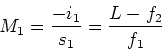(5)

The angular size of image produced by the compound microscope is,(6)

We also have M2 = h3/h2 = i2/s2 = i2/f2. We then have h3= i2 h2/f2. Note that we have omitted the minus signs in the calculation of magnification, so we have,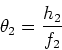(7)

Similarly, we have, M1 = h2/h1 = i1/s1 = (L-f2)/f1. Which implies that, h2 = (L-f2) h1/f1, so that,(8)

Finally, the angular magnification of a microscope is defined by,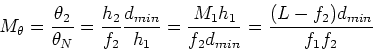(9)

Typical numbers are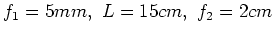, which gives a magnification of about 375.

Telescopes

The refracting telescope, probably first developed in Holland but Galileo built one of his own in 1609, consists of two converging lenses. As for the microscope, the first lens is the objective and the second is the eyepiece. The first lens takes the stars (sources at infinity) and forms an image, which is placed at the focal length of the eyepiece, which acts as a simple magnifier. The stars we wish to observe have an angular size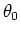. The image we observe in the eyepiece has angular extent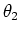. The focal lengths of the objective and eyepiece are f1 and f2 respectively. We have,(10)

Since h3/h2=i2/s2=i2/f2, we have h3 = h2 i2/f2, so that,(11)

Also h2/h1 = i1/s1, so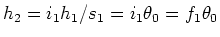, so we find,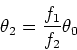(12)

The angular magnification of a telescope is then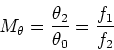(13)

The best magnification is found with large f1 and small f2. A second requirement is that the aperture be large enough so that enough light is focused for faint images to be visible. This is the main reason for building large telescopes.

The majority of modern telescopes are reflecting telescopes which work on the same principle except the objective is a large concave mirror. Newton is credited with developing the first reflecting telescope (1668).

Aberrations

There are many errors that can occur in telescopes and in microscopes. Monochromatic aberrations occur for light of one wavelength and include errors in machining the curvature of the lens (like the Hubble space telescope) and errors in focusing even if the lens was perfectly spherical (remember the small angle approximation we used?).

Chromatic aberrations occur in lenses due to the fact that the refractive index is different for different wavelengths. One of the advantages of the reflecting telescope is that mirrors do not suffer from chromatic aberration, though of course the eyepiece lens still does. Chromatic aberrations can be corrected using a second, diverging, lens that negates the chromatic aberrations of the converging lens system. Parabolic mirros can be designed to spherical aberrations. The final limiting factor is again diffraction effects due to the fact that light has a finite wavelength, as we shall see in a week or so.